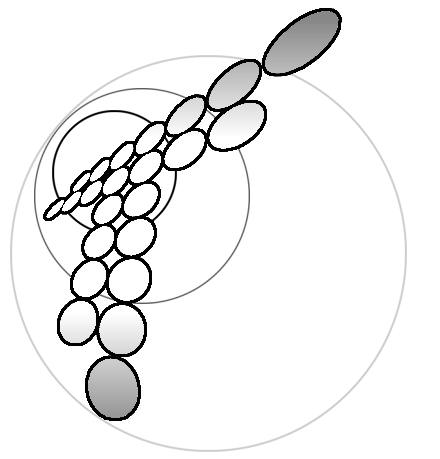# Generation of changing sing spase charge in a diffufion layer for electrode process with subsequent homogeneous reaction

```                             Yu.I.Kharkats, A.V.Sokirko
The A.N.Frumkin Institute of Electrochemistry,
Academy of Sciences of the USSR, Moscow
It is known that during electric  current  passing  a  space
charge region  arises  in  diffusion  layer,  whose  distribution
density decreases non-exponentially as it was in  diffuse  layer,
but in a sooth fashion . When the current density tends to its
limiting value the space charge density in the  vicinity  of  the
electrode  increases  abruptly  coinciding  in  sign  with   that
for electroactive ions.
Here we present the results of investigation of space charge
z+
distribution in diffusion layer for reduction of  cations  A    +
-     o                                              -        -
2e  -` A , and parallel oxygen reduction O  + 2H O + 4e  --` 4OH
2     2
-        +
with subsequent recombination reaction of  OH   and  H   ions  in
-    +
diffusion layer: OH  + H   --`  H O.  This  reaction  scheme  was
2
analyzed in detail  in  ref.  ,  in  which  the  mechanism  of
migration current  exaltation  effect  in  acidic  solutions  was
theoretically investigated.
The  set  of  electrodiffusion  equations   describing   the
distributions of component's concentrations and the potential  in
diffusion layer may be written as
dc        dJ    i  L       dc        dJ
1              1           2
--- + c  ---- = -----,     --- - c  ---- = 0,          (1)
1            0             2
dx        dx    FD c       dx        dx
1
dc        dJ           dc        dJ        i  L
3                      4                  2
D (--- - c  ---- ) - D  ( --- + c  ---- ) = - ---- = - D j ,  (2)
3        3           4          4               0      3 2
dx        dx           dx        dx         Fc
c c  = K ,      c  + c  = c  + c .              (3)
3 4    0        2    3    1    4
Here c , c , c  and c  - are dimensionless concentrations of
1   2   3      4
+            -           +
cations A , anions, OH  ions and H  ions, c  is  the  dimensional
0
+
concentration of A  ions in the solution bulk, J = Fv/RT  is  the
dimensionless electric potential, x is  dimensionless  coordinate
(0<x<1), L is the thickness of Nernst diffusion layer, i  and  i
1       2
are  current  densities  for  cation's   discharge   and   oxygen
reduction, K   -  the  ionic  product  for  water,  the  boundary
0
conditions at x=1 can be written as c (1)=1, c (1)=1+k,  c (1)=0,
1        2           3
c (1)=k, J(1)=0.
4
Taking into account that recombination reaction for water is
very fast and the equilibrium constant  satisfies  to  inequality
-
K , 1, we can suppose that in the diffusion layer either ions OH
0
+
or  H   ions  can  exist  simultaneously.  Correspondingly    the
recombination reaction plane x=q splits the diffusion layer  into
two regions 0<x<q and q<x<1. We can put  c >0  and  c ~0  in  the
4          3
region spaced to the right of the point x=q and c >0 and c ~0  in
3        4
the  region  spaced  to  the  left  of  the  point   x=0.   These
approximations  make  it  possible  to  determine  the  potential
distribution J(x) and then using Poisson equation to  find  space
charge distribution r = - e RT J"/F.
Calculated r(x) distributions for series of  parameters  j ,
1
and fixed j  and k values are shown in Figure.
2
Fig. Space charge distribution for D /D  = 0.56; k =  0.8;  j =4,
3  4                     2
0        2
and j : 1 - 2; 2 - 2.5; 3 - 2.7. r  = 2 e c  ( l /L) , l  is  the
1                            0             D       D
The  analysis  shows  that  as  a  result  of  recombination
reaction  inside  the  the  diffusion  layer   some   interesting
peculiarities in space charge distribution arise. At the point of
homogeneous reaction localization x=q  and  under  the  condition
D j  > kD j  the abrupt charge of the sign and absolute value  of
3 2     4 1
r(x) takes place. In the discussed model due  to  high  value  of
rate constant of recombination reaction its  reaction  layer  had
practically zero thickness. In  more  general  case  of  moderate
reaction rates there must exist some transition region  in  which
space  charge  varies   from   positive   to   negative   values.
Qualitatively the obtained result can be explained as follows. We
can present the plane of recombination reaction  localization  as
+
some electrode plane at x=q which is from fervor one side with H
-
ions and from the other side with OH  ions. Then  in  the  region
+
q<x<1 where H   ions  exist  we  have  as  in  usual  electrolyte
positive space charge distribution. And in the region 0<x<q where
-
OH   exist  we  have  correspondingly   negative   space   charge
distribution.
We note in  conclusion  that    the  peak  in  space  charge
distributions including also changing the sign  of  space  charge
was earlier predicted in refs.[3,4]  for  the  systems  in  which
besides movable carriers of charge also fixed charges exist.
References.
1. V.G.Levich. Physic-chemical Hydrodynamics.M.: 1959.
2. A.V.Sokirko, Yu.I.Kharkats, Electrokhimija, 25 (1989) 232.
3. Yu.I.Kharkats, Electrokhimija, 20 (1984) 248.
4.  Yu.Ya.Gurevich,  A.V.Noskov,  Yu.I.Kharkats,  Elekrokhimiya,
Proc. Acad. Sci. USSR, 298 (1988) 383.
```

Skin: